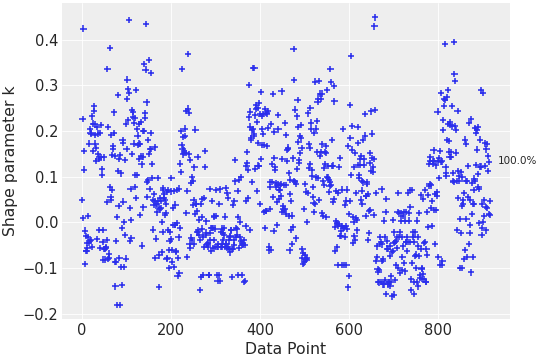# Pareto Shape Plot#

API documentation: `plot_khat()`Python source code: `[download source: matplotlib/mpl_plot_khat.py]`

```import matplotlib.pyplot as plt

import arviz as az

az.style.use("arviz-darkgrid")

loo = az.loo(idata, pointwise=True)

az.plot_khat(loo, show_bins=True)

plt.show()
```

Python source code: `[download source: bokeh/bokeh_plot_khat.py]`

```import arviz as az